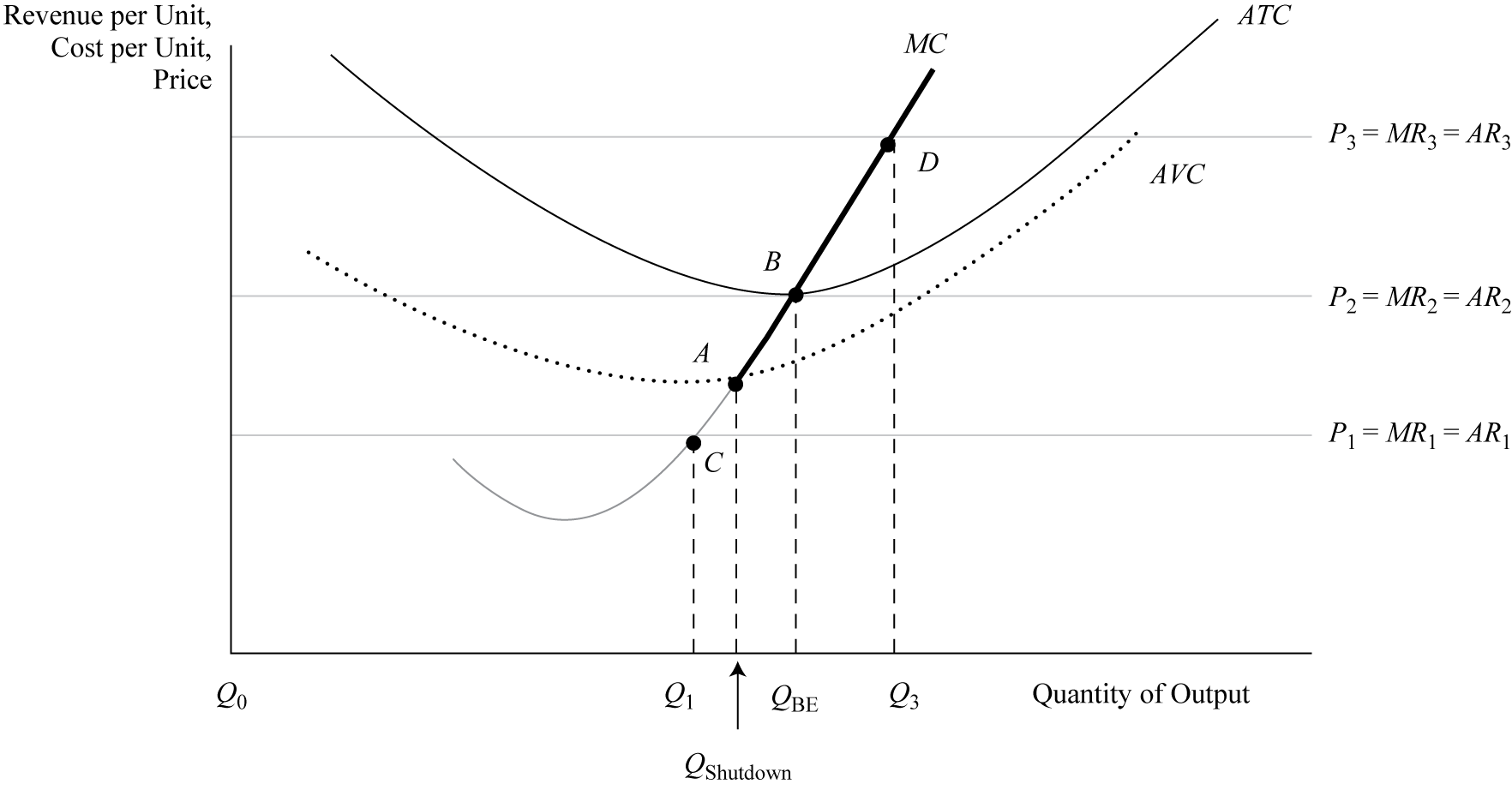#cfa #cfa-level-1 #economics #has-images #reading-15-demand-and-supply-analysis-the-firm #section-3-analysis-of-revenue-costs-and-profitExhibit 17 displays the firm’s supply curve, shutdown point, and breakeven level of operation under perfect competition in the short run. The firm’s short-run supply curve is the bold section of the marginal cost curve that lies above the minimum point (point A) on the average variable cost curve. If the firm operates below this point (for example between C and A), it shuts down because of its inability to cover variable costs in full. Between points A and B, the firm can operate in the short run because it is meeting variable cost payments even though it is unable to cover all of its fixed costs. In the long run, however, the firm is not able to survive if fixed costs are not completely covered. Any operating point above point B (the minimum point on ATC), such as point D, generates an economic profit.

A firm’s shutdown point occurs when average revenue is less than average variable cost (any output below Qshutdown), which corresponds to point A in Exhibit 17. Shutdown is defined as a situation in which the firm stops production but still confronts the payment of fixed costs in the short run as a business entity. In the short run, a business is capable of operating in a loss situation as long as it covers its variable costs even though it is not earning sufficient revenue to cover all fixed cost obligations. If variable costs cannot be covered in the short run (P < AVC), the firm will shut down operations and simply absorb the unavoidable fixed costs. This problem occurs at output Q1, which corresponds to point C where price is less than average variable cost. However, in the long run, to remain in business, the price must cover all costs. Therefore, in the long run, at any price below the breakeven point, the firm will exit the market, i.e., the firm will no longer participate in the market. Point D, which corresponds to output Q3, is a position where economic profit occurs because price is greater than ATC.

In the case of perfect competition, the breakeven point is the quantity where price, average revenue, and marginal revenue equal average total cost. It is also defined as the quantity where total revenue equals total costs. Firms strive to reach initial breakeven as soon as possible to avoid start-up losses for any extended period of time. When businesses are first established, there is an initial period where losses occur at low quantity levels. In Exhibit 17, the breakeven quantity occurs at output QBE, which corresponds to point B where price is tangent to the minimum point on the ATC. (Keep in mind that normal profit as an implicit cost is included in ATC as a fixed cost.)

Exhibit 18 shows the breakeven point under perfect competition using the total revenue–total cost approach. Actually, there are two breakeven points—lower (point E) and upper (point F). Below point E, the firm is losing money (economic losses), and beyond that point is the region of profitability (shaded area) that extends to the upper breakeven point. Within this profit area, a specific quantity (Qmax) maximizes profit as the largest difference between TR and TC. Point F is where the firm leaves the profit region and incurs economic losses again. This second region of economic losses develops when the firm’s production begins to reach the limits of physical capacity, resulting in diminished productivity and an acceleration of costs. Obviously, the firm would not produce beyond Qmax because it is the optimal production point that maximizes profit.

If you want to change selection, open original toplevel document below and click on "Move attachment"